•研究论文
• 基于CTI-EKF融合算法高效节能二次电池化成系统设计
• 原创 使用EKF融合odometry及imu数据 ...


原创                使用EKF融合odometry及imu数据

2018-05-22 11:11:04
cabinx
阅读数 11356

文章标签：
robot_pose_ekf                                                                    slam
更多

分类专栏：

slam

robot_pose_ekf

版权声明：本文为博主原创文章，遵循 CC 4.0 BY-SA 版权协议，转载请附上原文出处链接和本声明。

本文链接：https://blog.csdn.net/xiekaikaibing/article/details/80402113

整理资料发现早前学习robot_pose_ekf的笔记，大抵是一些原理基础的东西加一些自己的理解，可能有不太正确的地方。当时做工程遇到的情况为机器人在一些如光滑的地面上打滑的情形，期望使用EKF利用imu对odom数据进行校正。就结果来看，机器人旋转性能得到改善，前进方向性能没有改善，符合程序原理。若需要提高前进方向的性能，可以考虑加入VO或gps模块。实际工程中应注意odom数据进行协方差矩阵初始化的问题，否则程序会报错。
一、扩展卡尔曼滤波（EKF）原理
当前状态的概率的概率分布是关于上一状态和将要执行的控制量的二元函数，再叠加一个高斯噪声，测量值同样是关于当前状态的函数叠加高斯噪声。系统可为非线性系统。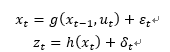对g(x_(t-1)，u_t )和h(x_t )泰勒展开，将其线性化，只取一次项为一阶EKF滤波。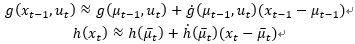g(xt-1,ut )在上一状态估计的最优值μt-1处取一阶导数，h(xt)在当前时刻预测值μ ̅t处取一阶导数，得到Gt和Ht。
结合通用的Kalman滤波公式如下：
预测过程：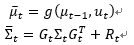更新过程：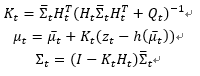二、卡尔曼滤波的多传感器融合
多传感器系统模型模型如下：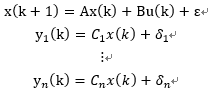和传统的卡尔曼滤波系统模型相比，多传感器系统的观测方程有多个，每个传感器的测量量都可以不同。
单观测模型系统：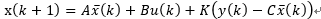卡尔曼滤波的核心为卡尔曼系数K的选取。对于多传感器系统，y(k)-Cx ̅(k)无法直接计算。
多传感器信息融合：
状态预测方程依然为：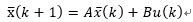第一个传感器的观测方程更新后得到系统的状态量x(k)及系统协方差矩阵Σ(k)。将二者作为下一个传感器更新过程的系统预测状态量x ̅(k)和系统预测协方差矩阵Σ ̅(k)进行状态更新。将最后一个传感器更新后得到的系统的状态量x(k)及系统协方差矩阵Σ(k)作为融合后输出，并将二者用于预测过程进行下一时刻的迭代。
扩展卡尔曼滤波工具包robot_pose_ekf
基于里程计系统建模：
系统状态量：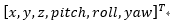系统输入：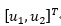系统方程：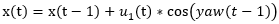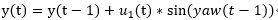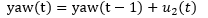过程激励协方差矩阵根据实际情况设定。同时在初始时刻，系统状态及系统协方差矩阵需要进行初始化。
观测系统模型：
通过topic信息分别得到轮式里程计（ODOMETRY）、IMU、视觉里程计(VO)、GPS各观测值和观测协方差矩阵，运用多传感器信息融合的原理对系统状态进行更新。
其中，ODOMETRY的观测量为[x,y,z,pitch,roll,raw]^T，观测矩阵为：
[1 0 0 0 0 0;0 1 0 0 0 0;0 0 0 0 0 0;0 0 0 0 0 0;0 0 0 0 0 0;0 0 0 0 0 0 1]
IMU的观测量为：[pitch,roll,raw]^T，观测矩阵为：
[0 0 0 1 0 0;0 0 0 0 1 0;0 0 0 0 0 1]
VO的观测量为[x,y,z,pitch,roll,raw]^T，观测矩阵为：
[1 0 0 0 0 0;0 1 0 0 0 0;0 0 1 0 0 0;0 0 0 1 0 0;0 0 0 0 1 0;0 0 0 0 0 0 1]
GPS的观测量为[x,y,z]^T，观测矩阵为：
[1 0 0 0 0 0;0 1 0 0 0 0;0 0 1 0 0 0]
三、程序流程（默认系统模型及各测量模型已建立完毕）：
①开始监听ODOMETRY、IMU、VO及GPS信息；
②构建扩展卡尔曼滤波器，具体可参考：https://blog.csdn.net/zhxue_11/article/details/83822625，扩展卡尔曼滤波器的非线性模型为上文提到的里程计模型；
③开始更新，起始只引入系统噪声；
④获取ODOMETRY信息作为观测量及观测协方差矩阵，进行状态更新，得到更新后的系统状态及系统协方差矩阵；
⑤获取IMU信息作为观测量及观测协方差矩阵，进行状态更新，得到更新后的系统状态及系统协方差矩阵；
⑥获取VO信息作为观测量及观测协方差矩阵，进行状态更新，得到更新后的系统状态及系统协方差矩阵；
⑦获取GPS信息作为观测量及观测协方差矩阵，进行状态更新，得到更新后的系统状态及系统协方差矩阵；
⑧发布更新后的状态量及协方差矩阵作为融合后的信息odom_combined。
四、robot_pose_ekf包使用方法
1、参数调整（odom_estimation_node.cpp中）：
①设定系统过程激励协方差矩阵；
②初始化设定系统协方差矩阵；
2、robot_pose_ekf.launch文件的设置：
①<param name="base_footprint_frame" value="base_footprint"/>将”base_footprint”替换为自身机器人坐标系；
②<param name="freq" value="30.0"/>EKF发布的频率为30Hz；
③<param name="sensor_timeout" value="1.0"/>传感器超时设置为1s；
④<param name="odom_used" value="true"/>启动轮式例程计信息作为观测量，”imu_used”，”vo_used”，”gps_used”同理；
⑤<remap from="odom" to="pr2_base_odometry/odom" />将”pr2_base_odometry/odom”替换为自身发布的odom的topic名称，该映射实现对自身机器人信息的监听，”imu”，”vo”，”gps”同理，注意其对应声明分别为“imu_data”、“vo”、“gps”。
五、robot_pose_ekf官方文档
http://wiki.ros.org/robot_pose_ekf?distro=kinetic
http://wiki.ros.org/robot_pose_ekf/Tutorials/AddingGpsSensor

文章最后发布于: 2018-05-22 11:11:04


展开全文• 本博文实现了通过EKF融合之前博客《ROS实验笔记之——基于ArUco Marker来估算camera的位姿》实现的odometry数据（实际上为marker定位的结果）以及IMUdata 源代码 注意这个包可能与“robot_localization-melodic-...
本博文实现了通过EKF融合之前博客《ROS实验笔记之——基于ArUco Marker来估算camera的位姿》实现的odometry数据（实际上为marker定位的结果）以及IMUdata

效果见如下视频

基于扩展卡尔曼滤波的Visual-marker与IMU融合定位

目录

源代码

测试

源代码

注意这个包可能与“robot_localization-melodic-devel”相冲突了，故此需要重命名一下或者把原来的删掉～

ekf_node.cpp（详细的注释见代码备注）

#include <iostream>
#include <ros/ros.h>
#include <ros/console.h>
#include <sensor_msgs/Imu.h>
#include <sensor_msgs/Range.h>
#include <nav_msgs/Odometry.h>
#include <Eigen/Eigen>

using namespace std;
using namespace Eigen;
ros::Publisher odom_pub;
MatrixXd Q = MatrixXd::Identity(12, 12);//预测的噪声
MatrixXd Rt = MatrixXd::Identity(6,6);//测量的噪声

//定义状态与协方差
VectorXd X_t=VectorXd::Zero(15);//类似于EKF包，
//x, y, z,
//roll, pitch, yaw,
//vx, vy, vz,
//vroll, vpitch, vyaw,
//ax, ay, az
MatrixXd Var_t = MatrixXd::Identity(15, 15);

//Rotation from the camera frame to the IMU frame
Eigen::Matrix3d Rcam;

//时间
double last_time=-1;
//重力加速度
double g=9.8;

//*********************************************************
//引入 inline 关键字的原因 在 c/c++ 中,为了解决一些频繁调用的小函数大量消耗栈空间(栈内存)的问题,
// 特别的引入了 inline 修饰符,表示为内联函数。
inline Eigen::Vector3d rotationMatrix2EulerVector_zxy(Eigen::Matrix3d R)
{
Eigen::Vector3d xyz_vector;
double phi = asinf64(R(2, 1));
double theta = atan2f64(-R(2, 0), R(2, 2));
double psi = atan2f64(-R(0, 1), R(1, 1));
xyz_vector << phi, theta, psi;

// Eigen::Vector3d zxy_vector = R.eulerAngles(2, 0, 1);
// Eigen::Vector3d temp;
// temp << zxy_vector(1), zxy_vector(2), zxy_vector(0);
// cout << "check eigen euler" << endl;
// cout << xyz_vector << ";" << temp << endl;
// xyz_vector <<
return xyz_vector;
}

inline Eigen::Matrix3d delGinv_delroll(double roll, double pitch)
{
// \frac{\partial G}{\partial \phi}
Eigen::Matrix3d deltaG;
double theta = pitch;
double phi = roll;
double cos2_1 = 1.0 / (cos(phi) * cos(phi) + 1e-8);
deltaG << 0, 0, 0,
sin(theta) * cos2_1, 0, -cos(theta) * cos2_1,
-sin(phi) * sin(theta) * cos2_1, 0, cos(theta) * sin(phi) * cos(phi);
return deltaG;
}

inline Eigen::Matrix3d delGinv_delpitch(double roll, double pitch)
{
// \frac{\partial G}{\partial \theta}
Eigen::Matrix3d deltaG;
double theta = pitch;
double phi = roll;
double cos_1 = 1.0 / (cos(phi) + 1e-8);
deltaG << -sin(theta), 0, cos(theta),
cos(theta) * sin(phi) * cos_1, 0, sin(theta) * sin(phi) * cos_1,
-cos(theta)* cos_1, 0 , - sin(theta) * cos_1;
return deltaG;
}

inline Eigen::Matrix3d deltaR_deltaroll(double roll, double pitch, double yaw)
{
// \frac{\partial R}{\partial \phi}
Eigen::Matrix3d deltaR;
double theta = pitch;
double phi = roll;
double psi = yaw;

deltaR <<
-cos(phi)*sin(psi)*sin(theta),  sin(phi)*sin(psi), cos(phi)*cos(theta)*sin(psi),
cos(phi)*cos(psi)*sin(theta), -cos(psi)*sin(phi), -cos(phi)*cos(psi)*cos(theta),
sin(phi)*sin(theta), cos(phi), -cos(theta)*sin(phi);

return deltaR;
}

inline Eigen::Matrix3d deltaR_deltapitch(double roll, double pitch, double yaw)
{
// \frac{\partial R}{\partial \phi}
Eigen::Matrix3d deltaR;
double theta = pitch;
double phi = roll;
double psi = yaw;

deltaR <<
- cos(psi)*sin(theta) - cos(theta)*sin(phi)*sin(psi), 0, cos(psi)*cos(theta) - sin(phi)*sin(psi)*sin(theta),
cos(psi)*cos(theta)*sin(phi) - sin(psi)*sin(theta), 0,  cos(theta)*sin(psi) + cos(psi)*sin(theta)*sin(phi),
-cos(phi)*cos(theta)                               , 0, -cos(phi)*sin(theta);
return deltaR;
}

inline Eigen::Matrix3d deltaR_deltayaw(double roll, double pitch, double yaw)
{
// \frac{\partial R}{\partial \phi}
Eigen::Matrix3d deltaR;
double theta = pitch;
double phi = roll;
double psi = yaw;

deltaR << - cos(theta)*sin(psi) - cos(psi)*sin(phi)*sin(theta), -cos(phi)*cos(psi), cos(psi)*cos(theta)*sin(phi) - sin(psi)*sin(theta),
cos(psi)*cos(theta) - sin(phi)*sin(psi)*sin(theta), -cos(phi)*sin(psi), cos(psi)*sin(theta) + sin(phi)*sin(psi)*cos(theta),
0, 0, 0;
return deltaR;
}
//*********************************************************

void publish_ekf_msg(VectorXd X, std_msgs::Header header){

// Eigen::Vector3d T_c_i;//camera position in the IMU frame
// T_c_i<<0.05, 0.05, 0;

// Matrix3d R1;//camera R in the world frame
// R1=AngleAxisd(X(5),Vector3d::UnitZ())*
//             AngleAxisd(X(3),Vector3d::UnitX())*
//             AngleAxisd(X(4),Vector3d::UnitY());
// //将IMU的结果转到world frame
// // R1.transposeInPlace();//原地转置
// Eigen::Matrix3d R_i_w=Rcam*R1;
// Eigen::Vector3d T_i_w=T_c_i+Rcam*X.head(3);

// Eigen::Matrix3d R_w_i=R_i_w.transpose();
// X.segment<3>(3)=rotationMatrix2EulerVector_zxy(R_w_i);
// X.segment<3>(0)=-R_w_i*T_i_w;

Eigen::Vector3d T_i_c;
T_i_c << 0.05, 0.05, 0;

Matrix3d R_1;
R_1 = AngleAxisd(X(5), Vector3d::UnitZ()) *
AngleAxisd(X(3), Vector3d::UnitX()) *
AngleAxisd(X(4), Vector3d::UnitY());
R_1.transposeInPlace();
Matrix3d R_cw = Rcam.transpose() * R_1;
X.segment<3>(3) = rotationMatrix2EulerVector_zxy(R_cw);
X.segment<3>(0) = Rcam.transpose() * (-R_1 * X.segment<3>(0) - T_i_c);

nav_msgs::Odometry new_msg;

double roll=X(3);
double pitch=X(4);
double yaw=X(5);
Matrix3d R;   //当前状态对应的旋转矩阵   旋转矩阵（3×3） Eigen::Matrix3d
R=AngleAxisd(yaw,Vector3d::UnitZ())*
AngleAxisd(roll,Vector3d::UnitX())*
AngleAxisd(pitch,Vector3d::UnitY());

Quaternion<double> q;
q=R;
new_msg.header=header;
new_msg.header.frame_id="world";
new_msg.pose.pose.position.x=X(0);
new_msg.pose.pose.position.y=X(1);
new_msg.pose.pose.position.z=X(2);
new_msg.pose.pose.orientation.w=q.w();
new_msg.pose.pose.orientation.x=q.x();
new_msg.pose.pose.orientation.y=q.y();
new_msg.pose.pose.orientation.z=q.z();
new_msg.twist.twist.linear.x=X(6);
new_msg.twist.twist.linear.y=X(7);
new_msg.twist.twist.linear.z=X(8);
new_msg.twist.twist.angular.x=X(9);
new_msg.twist.twist.angular.y=X(10);
new_msg.twist.twist.angular.z=X(11);

//x, y, z,
//roll, pitch, yaw,
//vx, vy, vz,
//vroll, vpitch, vyaw,
//ax, ay, az
odom_pub.publish(new_msg);

}

void imu_callback(const sensor_msgs::Imu::ConstPtr &msg)
{
//your code for propagation
//400HZ

//关于sensor message可以参考http://wiki.ros.org/sensor_msgs
//而其中的IMU在http://docs.ros.org/en/api/sensor_msgs/html/msg/Imu.html
//对于其中的stamp有注释如下：
// # Two-integer timestamp that is expressed as:
// # * stamp.sec: seconds (stamp_secs) since epoch (in Python the variable is called 'secs')
// # * stamp.nsec: nanoseconds since stamp_secs (in Python the variable is called 'nsecs')
// # time-handling sugar is provided by the client library
//那么定义的时间应该为：
double time=msg->header.stamp.sec+msg->header.stamp.nsec*1e-9; //单位为秒
double dt=0;
if (last_time>0){
dt=time-last_time;
}
last_time=time;

//IMU message：http://docs.ros.org/en/api/sensor_msgs/html/msg/Imu.html
//定义的消息如下：
// std_msgs/Header header
// geometry_msgs/Quaternion orientation
// float64 orientation_covariance
// geometry_msgs/Vector3 angular_velocity
// float64 angular_velocity_covariance
// geometry_msgs/Vector3 linear_acceleration
// float64 linear_acceleration_covariance

Vector3d omega_imu;//角速度
omega_imu<<msg->angular_velocity.x,msg->angular_velocity.y,msg->angular_velocity.z;
// omega_m=msg->angular_velocity; (没有这个运算符)

Vector3d a_imu;//线加速度
a_imu<<msg->linear_acceleration.x,msg->linear_acceleration.y,msg->linear_acceleration.z;

double roll=X_t(3);
double pitch=X_t(4);
double yaw=X_t(5);
//Rotation Matrix
Matrix3d R;   //当前状态对应的旋转矩阵   旋转矩阵（3×3） Eigen::Matrix3d
//旋转向量（3×1）Eigen::AngleAxisd
//AngleAxisd(yaw,Vector3d::UnitZ())初始化旋转向量，角度为yaw，旋转轴为Z
//**********************
// 欧拉角转到旋转矩阵的做法：
// Eigen::AngleAxisd rollAngle(AngleAxisd(eulerAngle(2),Vector3d::UnitX()));
// Eigen::AngleAxisd pitchAngle(AngleAxisd(eulerAngle(1),Vector3d::UnitY()));
// Eigen::AngleAxisd yawAngle(AngleAxisd(eulerAngle(0),Vector3d::UnitZ()));

// Eigen::Matrix3d rotation_matrix;
// rotation_matrix=yawAngle*pitchAngle*rollAngle;
//**********************
R=AngleAxisd(yaw,Vector3d::UnitZ())*AngleAxisd(roll,Vector3d::UnitX())*AngleAxisd(pitch,Vector3d::UnitY());

//状态的变化
VectorXd dotX_t=VectorXd::Zero(15);
dotX_t.head(3)=X_t.segment<3>(6);//参考：https://blog.csdn.net/shuzfan/article/details/52367329
//x.head<n>()// x(1:n)
// x.segment<n>(i)// x(i+1 : i+n)   X_t(6:9)为//vx, vy, vz,

//x, y, z,
//roll, pitch, yaw,
//vx, vy, vz,
//vroll, vpitch, vyaw,
//ax, ay, az

//angular velocity change （角速度的变化）
//angular velocity in the body frame (IMU) =matrix (G) * world angular velocity
// /matrix (G)为：
Matrix3d G, G_inverse;
G<<cos(pitch),0,  -cos(roll)*sin(pitch),
0,        1,   sin(roll),
sin(pitch),0,   cos(roll)*cos(pitch);

G_inverse=G.inverse();
dotX_t.segment<3>(3)=G_inverse*(omega_imu-X_t.segment<3>(9));
dotX_t.segment<3>(6)=R*(a_imu-X_t.segment<3>(12));
dotX_t(8)=dotX_t(8)+g;

//计算状态转移矩阵的雅可比矩阵
MatrixXd F=MatrixXd::Identity (15,15);

// Derivatives for G, R
MatrixXd dGinv_droll    = delGinv_delroll(roll, pitch);
MatrixXd dGinv_dpitch   = delGinv_delpitch(roll, pitch);
MatrixXd dR_droll       = deltaR_deltaroll(roll, pitch, yaw);
MatrixXd dR_dpitch      = deltaR_deltapitch(roll, pitch, yaw);
MatrixXd dR_dyaw        = deltaR_deltayaw(roll, pitch, yaw);

F.block<3, 3>(0, 6) = MatrixXd::Identity(3, 3) * dt; // position -> speed

F.block<3, 3>(3, 9) -= G_inverse * dt; // orientation -> bg
F.block<3, 1>(3, 3) += dGinv_droll  * (omega_imu - X_t.segment<3>(9)) * dt; // orientation -> roll
F.block<3, 1>(3, 4) += dGinv_dpitch * (omega_imu - X_t.segment<3>(9)) * dt; // orientation -> pitch

F.block<3, 1>(6, 3) += dR_droll * (a_imu - X_t.segment<3>(12)) * dt; // velocity -> roll/pitch/yaw
F.block<3, 1>(6, 4) += dR_dpitch * (a_imu - X_t.segment<3>(12)) * dt;
F.block<3, 1>(6, 5) += dR_dyaw * (a_imu - X_t.segment<3>(12)) * dt;
F.block<3, 3>(6, 12) -= R * dt;

MatrixXd U=MatrixXd::Identity (15,12);
//P.block<rows, cols>(i, j) // P(i+1 : i+rows, j+1 : j+cols)
U.block<3,3>(3,0)=G_inverse;  //U(3+1:3+3. 0+1:0+3)
U.block<3,3>(6,3)=R;
MatrixXd V=MatrixXd::Identity (15,12);
V=U*dt;

//EKF的状态预测
X_t=X_t+dt*dotX_t;
Var_t=F*Var_t*F.transpose()+V*Q*V.transpose();
//需要将当前的预测X_t发布嘛？？？
//在此处发布而不在measurement处发布的原因在于此次的频率更高，而measurement会自动更新状态
publish_ekf_msg(X_t,msg->header);
}

void odom_callback(const nav_msgs::Odometry::ConstPtr &msg)   //marker
{
//your code for update
// camera position in the IMU frame = (0.05, 0.05, 0)
// camera orientaion in the IMU frame = Quaternion(0, 1, 0, 0); w x y z, respectively
//					   RotationMatrix << 1, 0, 0,
//							             0, -1, 0,
//                                       0, 0, -1;

//20 HZ

//get measurements
Eigen::Matrix3d R_c_w;//camera在world frame下的旋转矩阵
Eigen::Vector3d T_c_w;
//赋值
T_c_w<<msg->pose.pose.position.x,msg->pose.pose.position.y,msg->pose.pose.position.z;
Eigen::Quaterniond q(
msg->pose.pose.orientation.w,
msg->pose.pose.orientation.x,
msg->pose.pose.orientation.y,
msg->pose.pose.orientation.z
);
R_c_w=q;

//Transform back
Eigen::Vector3d T_c_i;//camera position in the IMU frame
T_c_i<<0.05, 0.05, 0;

//IMU在world frame下的pose
Eigen::Matrix3d R_i_w=Rcam*R_c_w;
Eigen::Vector3d T_i_w=T_c_i+Rcam*T_c_w;

Eigen::Matrix3d R_w_i=R_i_w.transpose();
Eigen::Vector3d euler_meas=rotationMatrix2EulerVector_zxy(R_w_i);
Eigen::Vector3d T_w_i=-R_w_i*T_i_w;

VectorXd measurement_different(6);
measurement_different.head(3)=T_w_i-X_t.head(3);
measurement_different.segment<3>(3)=euler_meas-X_t.segment<3>(3);
//对角度进行归一化到-pi~pi区间
for (int i=3;i<6;i++){
if (measurement_different(i)>M_PI){
measurement_different(i)=measurement_different(i)-2*M_PI;
}
else if(measurement_different(i)<-M_PI){
measurement_different(i)=measurement_different(i)+2*M_PI;
}
}

Eigen::MatrixXd C=Eigen::MatrixXd::Zero(6,15);
C.block<6,6>(0,0)=Eigen::MatrixXd::Identity(6,6);
Eigen::MatrixXd W=Eigen::MatrixXd::Identity(6,6);

Eigen::MatrixXd K=Var_t*C.transpose()*(C*Var_t*C.transpose()+W*Rt*W.transpose()).inverse();

//measuremnet update
X_t=X_t+K*measurement_different;
Var_t=Var_t-K*C*Var_t;

for (int i=3;i<6;i++){
if (X_t(i)>M_PI){
X_t(i)=X_t(i)-2*M_PI;
}
else if(X_t(i)<-M_PI){
X_t(i)=X_t(i)+2*M_PI;
}
}

}

int main(int argc, char **argv)
{
ros::init(argc, argv, "ekf");
ros::NodeHandle n("~");
ros::Subscriber s1 = n.subscribe("imu", 1000, imu_callback);//做预测
ros::Subscriber s2 = n.subscribe("tag_odom", 1000, odom_callback);//做测量更新，虽然称呼为odom，但实际上是基于PNP的3D-2D pose estimation
odom_pub = n.advertise<nav_msgs::Odometry>("ekf_odom", 100);
Rcam = Quaterniond(0, 1, 0, 0).toRotationMatrix();//camera orientaion in the IMU frame
cout << "R_cam" << endl << Rcam << endl;

// Q imu covariance matrix; Rt visual odomtry covariance matrix
// You should also tune these parameters
// Q.topLeftCorner(6, 6) = 0.01 * Q.topLeftCorner(6, 6);
// Q.bottomRightCorner(6, 6) = 0.01 * Q.bottomRightCorner(6, 6);
// Rt.topLeftCorner(3, 3) = 0.1 * Rt.topLeftCorner(3, 3);
// Rt.bottomRightCorner(3, 3) = 0.1 * Rt.bottomRightCorner(3, 3);
// Rt.bottomRightCorner(1, 1) = 0.1 * Rt.bottomRightCorner(1, 1);

Var_t.block<6, 6>(9, 9) = MatrixXd::Identity(6, 6) * 0.01;

Q.topLeftCorner(6, 6) = 0.1 * Q.topLeftCorner(6, 6);
Q.bottomRightCorner(6, 6) = 0.005 * Q.bottomRightCorner(6, 6);
Rt.topLeftCorner(3, 3) = 0.1 * Rt.topLeftCorner(3, 3);
Rt.bottomRightCorner(3, 3) = 0.1 * Rt.bottomRightCorner(3, 3);
Rt.bottomRightCorner(1, 1) = 0.1 * Rt.bottomRightCorner(1, 1);

ros::spin();
}


对应的launch文件

<launch>

<!-- <node name="rosbag" pkg="rosbag" type="play" args=" $(find ekf)/bag/ekf_A3.bag -r 1" /> --> <node name="rosbag" pkg="rosbag" type="play" args="$(find ekf)/bag/ekf_A3.bag -r 0.5" />
<!-- 更改了视频播放的速率 -->
<node pkg="ekf" type="ekf" name="ekf" output="screen">
<remap from="~imu" to="/djiros/imu"/>
<!-- <remap from="~tag_odom" to="/tag_detector/odom_yourwork"/> -->
<remap from="~tag_odom" to="/tag_detector/odom_ref"/>
</node>

<node pkg="tag_detector" type="tag_detector" name="tag_detector" output="log">
<remap from="~image_raw" to="/djiros/image"/>
<param name="cam_cal_file" type="string" value="$(find ekf)/config/TA-camera.yml"/> <param name="board_config_file" type="string" value="$(find ekf)/config/tag_1.yml"/>
</node>

</launch>

测试

运行代码是

roslaunch ekf A3.launch 

注意，对于launch如果不小心用了rosrun运行，会出现下面的错误：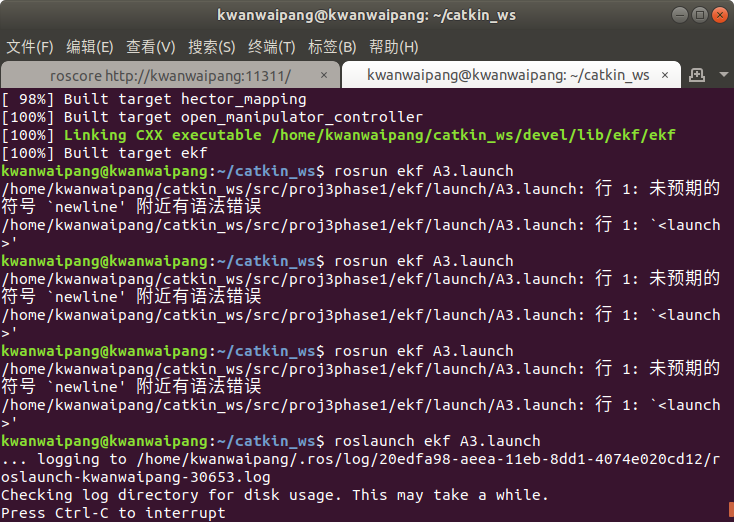通过rviz与rqt_plot来可视化结果～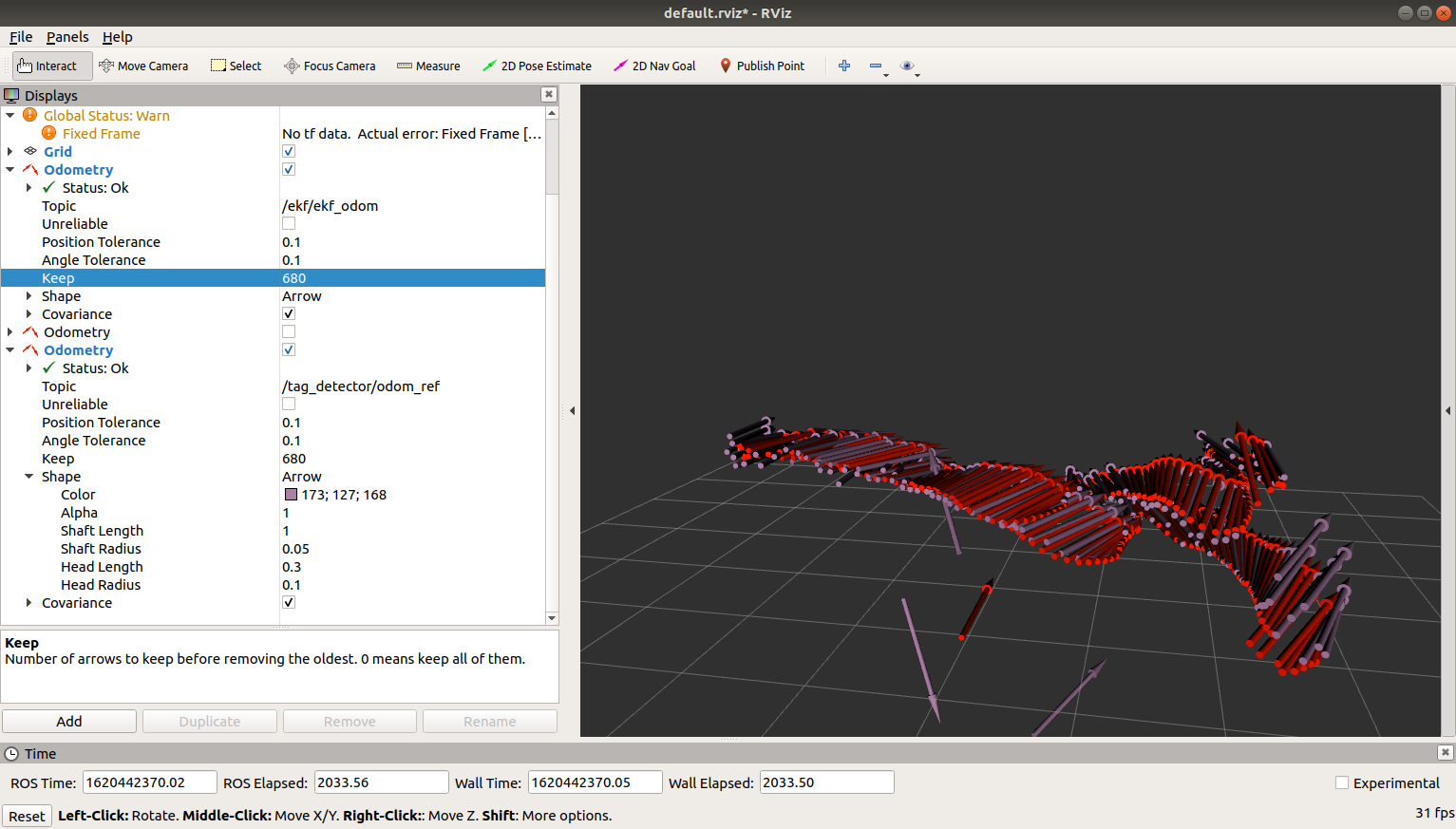更多实验的效果可见视频～


展开全文• 1.这里是ros_ekf_pose包的简单介绍: 这个包用于评估机器人的3D位姿，使用了来自不同源的位姿测量信息，它... 基本思路就是用松耦合方式融合不同传感器信息实现位姿估计。   1 如何使用EKF 1.1 配置    E...
1.这里是ros_ekf_pose包的简单介绍:

这个包用于评估机器人的3D位姿，使用了来自不同源的位姿测量信息，它使用带有6D（3D position and 3D orientation）模型信息的扩展卡尔曼滤波器来整合来自轮子里程计，IMU传感器和视觉里程计的数据信息。 基本思路就是用松耦合方式融合不同传感器信息实现位姿估计。

1 如何使用EKF

1.1 配置

EKF node的缺省启动文件位于robot_pose_ekf包中，文件中有许多配置参数：

freq: 滤波器更新和发布频率，注意：频率高仅仅意味着一段时间可以获得更多机器人位姿信息，但是并不表示可以提高每次位姿评估的精度
sensor_timeout: 当某传感器停止给滤波器发送信息时，滤波器应该等多长时间方才可以在没有该传感器信息状况下继续工作
odom_used, imu_used, vo_used: enable/disable输入源
启动文件中配置参数设置可以被修改，看起来大致如下所示：

<launch>
<node pkg="robot_pose_ekf" type="robot_pose_ekf" name="robot_pose_ekf">
<param name="output_frame" value="odom"/>
<param name="freq" value="30.0"/>
<param name="sensor_timeout" value="1.0"/>
<param name="odom_used" value="true"/>
<param name="imu_used" value="true"/>
<param name="vo_used" value="true"/>
<param name="debug" value="false"/>
<param name="self_diagnose" value="false"/>
</node>
</launch>

1.2 编译并运行该包

Build the package

 $rosdep install robot_pose_ekf$ roscd robot_pose_ekf
$rosmake Run the robot pose ekf $ roslaunch robot_pose_ekf.launch

2 Nodes

2.1 robot_pose_ekf

为确定机器人位姿，robot_pose_ekf包实现了扩展卡尔曼滤波器

2.1.1 Subscribed Topics

odom (nav_msgs/Odometry)

2D pose (used by wheel odometry): 该2D pose包含了机器人在地面的位置（position）和方位（orientation）信息以及该位姿的协方差（covariance）。
用来发送该2D位姿的消息实际上表示了一个3D位姿，只不过把z，pitch和roll分量简单忽略了。
imu_data (sensor_msgs/Imu)

3D orientation (used by the IMU): 3D方位提供机器人底座相对于世界坐标系的Roll, Pitch and Yaw偏角。 Roll and Pitch角是绝对角度（因为IMU使用了重力参考），而YAW角是相对角度。 协方差矩阵用来指定方位测量的不确定度。当仅仅收到这个主题消息时，机器人位姿ekf还不会启动，因为它还需要来自主题'vo'或者'odom'的消息。
vo (nav_msgs/Odometry)

3D pose (used by Visual Odometry): 3D位姿可以完整表示机器人的位置和方位并给出位姿协方差。当用传感器只测量部分3D位姿(e.g. the wheel odometry only measures a 2D pose)时候, 可以给还未真正开始测量的部分3D位姿先简单指定一个大的协方差。
robot_pose_ekf node并不需要所有3个传感器源一直同时可用。 每个源都能提供位姿和协方差，且这些源以不同速率和延时工作。 随着时间推移某个源可能出现和消失，该node可以自动探测当前可用的源。 如果要把自己想使用的传感器加入到输入源中，请参考指南  the Adding a GPS sensor tutorial。

2.1.2 Published Topics

robot_pose_ekf/odom_combined (geometry_msgs/PoseWithCovarianceStamped)

滤波器输出 (估计的3D机器人位姿)

2.1.3 Provided tf Transforms

odom_combined → base_footprint

3 EKF如何工作

3.1 Pose interpretation

给滤波器node提供信息的所有传感器源都有自己的参考坐标系，并且随着时间推移都可能出现漂移现象。因此，每个传感器发出来的绝对位姿不能直接对比。 因此该node使用每个传感器的相对位姿差异来更新扩展卡尔曼滤波器。

3.2 Covariance interpretation

当机器人在四周移动时候，随着时间推移位姿不确定性会变得越来越大，协方差会无边界的增长。这样一来发布位姿自身绝对协方差没有意义，因此传感器源会发布一段时间协方差的变化（例如，速速协方差）。

3.3 Timing

假定机器人上次更新位姿滤波器在t_0时刻， 该node只有在收到每个传感器测量值（时间戳>t_0）之后才会进行下一次的滤波器更新。 例如，在odom topic收到一条消息时间戳（t_1 > t_0）, 且在imu_data topic上也收到一条消息（ 其时间戳t_2 > t_1 > t_0）, 滤波器将被更新到所有传感器信息可用的最新时刻，这个时刻是t_1。 在t_1时刻odom位姿直接给出了，但是imu位姿需要通过在t_0和t_2两时刻之间进行线性插值求得。 在t_0到 t_1时间段，机器人位姿滤波器使用odom和IMU相对位姿进行更新。上图是PR2机器人机器人的实验结果显示，从绿色初始位置开始移动最后回到出发位置。 完美的odometry x-y曲线图应该是一个精确的闭环曲线图。 上图蓝色线是轮式里程计的输入，蓝色点是其估计的结束位置。红色线是robot_pose_ekf的输出, robot_pose_ekf整合了轮式里程计和IMU的信息，给出了红色的结束位置点。

4 Package Status

4.1 Stability

该包的基础部分代码已经被充分测试且稳定较长时间。 但是ROS API一直在随着消息类型的变化在被升级。

4.2 Roadmap

目前该滤波器设计被用于在PR2上使用的三种传感器 (wheel odometry, imu and vo)。 下一步计划是让该包更加通用可以监听更多传感器源，所有发布均使用消息 (nav_msgs/Odometry) 。

增加速度到扩展卡尔曼滤波器状态中

5 Tutorials

robot_pose_ekf/Tutorials/AddingGpsSensor
2. 简单介绍协方差:转载http://blog.sina.com.cn/s/blog_4aa4593d01012am3.html

今天看论文的时候又看到了协方差矩阵这个破东西，以前看模式分类的时候就特困扰，没想到现在还是搞不清楚，索性开始查协方差矩阵的资料，恶补之后决定马上记录下来，嘿嘿~本文我将用自认为循序渐进的方式谈谈协方差矩阵。

统计学的基本概念

学过概率统计的孩子都知道，统计里最基本的概念就是样本的均值，方差，或者再加个标准差。首先我们给你一个含有n个样本的集合，依次给出这些概念的公式描述，这些高中学过数学的孩子都应该知道吧，一带而过。均值：标准差：方差：很 显然，均值描述的是样本集合的中间点，它告诉我们的信息是很有限的，而标准差给我们描述的则是样本集合的各个样本点到均值的距离之平均。以这两个集合为 例，[0，8，12，20]和[8，9，11，12]，两个集合的均值都是10，但显然两个集合差别是很大的，计算两者的标准差，前者是8.3，后者是 1.8，显然后者较为集中，故其标准差小一些，标准差描述的就是这种“散布度”。之所以除以n-1而不是除以n，是因为这样能使我们以较小的样本集更好的 逼近总体的标准差，即统计上所谓的“无偏估计”。而方差则仅仅是标准差的平方。

为什么需要协方差？

上 面几个统计量看似已经描述的差不多了，但我们应该注意到，标准差和方差一般是用来描述一维数据的，但现实生活我们常常遇到含有多维数据的数据集，最简单的 大家上学时免不了要统计多个学科的考试成绩。面对这样的数据集，我们当然可以按照每一维独立的计算其方差，但是通常我们还想了解更多，比如，一个男孩子的 猥琐程度跟他受女孩子欢迎程度是否存在一些联系啊，嘿嘿~协方差就是这样一种用来度量两个随机变量关系的统计量，我们可以仿照方差的定义：来度量各个维度偏离其均值的程度，标准差可以这么来定义：协方差的结果有什么意义呢？如果结果为正值，则说明两者是正相关的(从协方差可以引出“相关系数”的定义)，也就是说一个人越猥琐就越受女孩子欢迎，嘿嘿，那必须的~结果为负值就说明负相关的，越猥琐女孩子越讨厌，可能吗？如果为0，也是就是统计上说的“相互独立”。

从协方差的定义上我们也可以看出一些显而易见的性质，如：协方差多了就是协方差矩阵

上一节提到的猥琐和受欢迎的问题是典型二维问题，而协方差也只能处理二维问题，那维数多了自然就需要计算多个协方差，比如n维的数据集就需要计算个协方差，那自然而然的我们会想到使用矩阵来组织这些数据。给出协方差矩阵的定义：这个定义还是很容易理解的，我们可以举一个简单的三维的例子，假设数据集有三个维度，则协方差矩阵为可见，协方差矩阵是一个对称的矩阵，而且对角线是各个维度上的方差。

Matlab协方差实战

上面涉及的内容都比较容易，协方差矩阵似乎也很简单，但实战起来就很容易让人迷茫了。必须要明确一点，协方差矩阵计算的是不同维度之间的协方差，而不是不同样本之间的。这个我将结合下面的例子说明，以下的演示将使用Matlab，为了说明计算原理，不直接调用Matlab的cov函数(蓝色部分为Matlab代码)。

首先，随机产生一个10*3维的整数矩阵作为样本集，10为样本的个数，3为样本的维数。
MySample = fix(rand(10,3)*50)根据公式，计算协方差需要计算均值，那是按行计算均值还是按列呢，我一开始就老是困扰这个问题。前面我们也特别强调了，协方差矩阵是计算不同维度间的协方差，要时刻牢记这一点。样本矩阵的每行是一个样本，每列为一个维度，所以我们要按列计算均值。为了描述方便，我们先将三个维度的数据分别赋值：

dim1 = MySample(:,1);
dim2 = MySample(:,2);
dim3 = MySample(:,3);

计算dim1与dim2，dim1与dim3，dim2与dim3的协方差：

sum( (dim1-mean(dim1)) .* (dim2-mean(dim2)) ) / ( size(MySample,1)-1 ) % 得到  74.5333
sum( (dim1-mean(dim1)) .* (dim3-mean(dim3)) ) / ( size(MySample,1)-1 ) % 得到  -10.0889
sum( (dim2-mean(dim2)) .* (dim3-mean(dim3)) ) / ( size(MySample,1)-1 ) % 得到  -10***000

搞清楚了这个后面就容易多了，协方差矩阵的对角线就是各个维度上的方差，下面我们依次计算：

std(dim1)^2 % 得到   108.3222
std(dim2)^2 % 得到   260.6222
std(dim3)^2 % 得到  94.1778

这样，我们就得到了计算协方差矩阵所需要的所有数据，调用Matlab自带的cov函数进行验证：

cov(MySample)把我们计算的数据对号入座，是不是一摸一样？

Update： 今天突然发现，原来协方差矩阵还可以这样计算，先让样本矩阵中心化，即每一维度减去该维度的均值，使每一维度上的均值为0，然后直接用新的到的样本矩阵乘 上它的转置，然后除以(N-1)即可。其实这种方法也是由前面的公式通道而来，只不过理解起来不是很直观，但在抽象的公式推导时还是很常用的！同样给出 Matlab代码实现：

X = MySample – repmat(mean(MySample),10,1);    % 中心化样本矩阵，使各维度均值为0
C = (X’*X)./(size(X,1)-1)

总结

理解协方差矩阵的关键就在于牢记它计算的是不同维度之间的协方差，而不是不同样本之间，拿到一个样本矩阵，我们最先要明确的就是一行是一个样本还是一个维度，心中明确这个整个计算过程就会顺流而下，这么一来就不会迷茫了~

P.S.写论文要选Latex，在wordpress里编辑公式还得用Latex，用Latex还真对得起咱学计算机这张脸~

例2：

a =
-1     1     2
-2     3     1
4     0     3
for i=1:size(a,2)
for j=1:size(a,2)
c(i,j)=sum((a(:,i)-mean(a(:,i))).*(a(:,j)-mean(a(:,j))))/(size(a,1)-1);
end
end

c =

10.3333   -4.1667    3.0000

-4.1667    2.3333   -1.5000

3.0000   -1.5000    1.0000

c为求得的协方差矩阵，在matlab以矩阵a的每一列为变量，对应的每一行为样本。这样在矩阵a中就有3个列变量分别为a（:,1), a(:,2), a(:,3)。

在协方差矩阵c中，每一个元素c(i,j)为对第i列与第j列的协方差，例如c(1,2) = -4.1667为第一列与第二列的协方差。

拿c(1,2)的求解过程来说

c(1,2)=sum((a(:,1)-mean(a(:,1))).*(a(:,2)-mean(a(:,2))))/(size(a,1)-1);

1. a(:,1)-mean(a(:,1))，第一列的元素减去该列的均值得到

-1.3333

-2.3333

3.6667

2,  a(:,2)-mean(a(:,2))，第二列的元素减去该列的均值得到

-0.3333

1.6667

-1.3333

3, 再将第一步与第二部的结果相乘

-1.3333        -0.3333           0.4444

-2.3333  .*     1.6667  =     -3.8889

3.6667         -1.3333          -4.8889

4, 再将结果求和/size(a,1)-1 得 -4.1667,该值即为c（1,2）的值。

再细看一下是不是与协方差公式：Cov(X,Y) = E{ [ (X-E(X) ] [ (Y-E(Y) ] } 过程基本一致呢，只是在第4步的时候matlab做了稍微的调整，自由度为n-1，减少了一行的样本值个数。

转载:https://blog.csdn.net/xiekaikaibing/article/details/80403264?utm_source=blogxgwz2

在使用robot_pose_ekf时，常遇到接收到的odometry数据格式错误的问题。一个可能的原因为底盘或其他设备发布odometry数据的协方差矩阵默认为0矩阵。解决的方法由两种：一种为在底盘将信息封装发布前对协方差矩阵进行初始化；另一种方法为在robot_pose_ekf中添加判断，如果接收到的odometry信息的协方差矩阵没有进行初始化，则进行初始化。

做工程时采用了第二种方法。初始化位于odom_estimation_node.cpp中：

void OdomEstimationNode::odomCallback(const OdomConstPtr& odom)
{
...
odom_meas_  = Transform(q, Vector3(odom->pose.pose.position.x, odom->pose.pose.position.y, 0));
for (unsigned int i=0; i<6; i++)
for (unsigned int j=0; j<6; j++)
odom_covariance_(i+1, j+1) = odom->pose.covariance[6*i+j];

if (odom_covariance_(1,1) == 0.0){
SymmetricMatrix measNoiseOdom_Cov(6);  measNoiseOdom_Cov = 0;
measNoiseOdom_Cov(1,1) = pow(0.01221,2);  // = 0.01221 meters / sec
measNoiseOdom_Cov(2,2) = pow(0.01221,2);  // = 0.01221 meters / sec
measNoiseOdom_Cov(3,3) = pow(0.01221,2);  // = 0.01221 meters / sec
measNoiseOdom_Cov(4,4) = pow(0.007175,2);  // = 0.41 degrees / sec
measNoiseOdom_Cov(5,5) = pow(0.007175,2);  // = 0.41 degrees / sec
measNoiseOdom_Cov(6,6) = pow(0.007175,2);  // = 0.41 degrees / sec
odom_covariance_ = measNoiseOdom_Cov;
}

my_filter_.addMeasurement(StampedTransform(odom_meas_.inverse(), odom_stamp_, base_footprint_frame_, "wheelodom"), odom_covariance_);

...
}

由于对于我手头情况而言odometry确定协方差矩阵为0，故判断条件设得较为简单：odom_covariance_(1,1) == 0.0。判断条件应根据实际情况设定。协方差矩阵具体值可以考虑设置为精度的二次方。

关于卡尔曼滤波中协方差矩阵的设定没能深入学习理解，感觉较为复杂。但在做工程中确保odom的协方差矩阵对角线元素不均0则robot_pose_ekf即可工作。

展开全文• robot_pose_ekf.launch 默认配置： 2d odom imu vo                       2.1.1 订阅主题 odom（编码器） (nav_msgs/Odometry)2D po
robot_pose_ekf.launch  默认配置： 2d  odom   imu  vo
<launch>
<node pkg="robot_pose_ekf" type="robot_pose_ekf" name="robot_pose_ekf">
<param name="output_frame" value="odom_combined"/>
<param name="base_footprint_frame" value="base_footprint"/>
<param name="freq" value="30.0"/>
<param name="sensor_timeout" value="1.0"/>
<param name="odom_used" value="true"/>
<param name="imu_used" value="true"/>
<param name="vo_used" value="true"/>
<remap from="odom" to="pr2_base_odometry/odom" />
</node>    </launch>

2.1.1 订阅主题

odom（编码器）
(nav_msgs/Odometry)2D pose (轮式里程计): 二维姿态包含机器人平面中的坐标和朝向以及方位协方差。平面机器人中，其中z, roll and pitch 忽略。
imu_data（IMU）
(sensor_msgs/Imu)3D orientation (used by the IMU): 提供包含相对世界坐标系的Roll, Pitch 和 Yaw 角度。 Roll 和 Pitch 角是绝对角度，Yaw是相对角度。协方差矩阵决定了方向的不确定性， robot pose
ekf 在仅仅接收此msg时不会工作，它需要 'vo' 或'odom' 主题。
vo（视觉里程计）
(nav_msgs/Odometry)3D pose (used by Visual Odometry): 此主题包含机器人全方向及相应协方差信息，当传感器只测量部分3维信息时，需要制定一个较大的协方差用来忽略此项数据。
The robot_pose_ekf节点不需要三个主题同时有效，每个主题数据都会产生一个位置估计及协方差。主题的频率也不一定一致，也具有不同的延迟。数据间歇收发，这时节点将自动检测使用有效的传感数据。添加自主的传感数据参照GPS的示例即可。 http://wiki.ros.org/robot_pose_ekf/Tutorials/AddingGpsSensor

2.1.2 发布的主题

robot_pose_ekf/odom_combined (geometry_msgs/PoseWithCovarianceStamped)
《滤波器的输出 (the estimated 3D robot pose)》
2.1.3 TF变换
odom_combined → base_footprint\

3. Robot Pose EKF如何工作
3.1 位置解析

所有传感器发送的数据依据自己的世界坐标系，每个世界坐标系将随着时间漂移，因此，各个传感器发送的绝对位置无法直接比较，节点使用每个传感器的相对坐标系更新滤波器。
3.2 协方差解析
随着机器人行动，机器人全局位置的不确定性越来越大，协方差也将逐渐增大而无界限。因此在位置本身发布协方差是毫无意义的，取而代之的是传感器数据发布随时间变化的协方差， *注意通过外部传感器观测将减少估计的不确定性，不过这是定位技术而不是轨迹推演。
3.3 Timing时间
假设滤波器上一刻在t_0时间更新，直到某一传感器数据的时间戳在t_0之后获取，滤波器才会工作。. The node will not update the robot pose filter until at least one measurement ofeach sensor arrived with a timestamp later than t_0. When e.g. a message was 当一个odom传感器接受时间 t_1 > t_0，IMU的数据时间戳在t_2
> t_1 > t_0，在上一刻所有传感器数据都就绪的时间戳滤波器将会更新。本例在 t_1具有odom数据而IMU数据是t_0 到 t_2间线性插入的，滤波器将会更新t_0 到 t_1间的相对odom和IMU数据。上图所示，PR2在给定的起点（绿色）开始，里程计图展示了整个闭环，蓝色线表示编码器的输入，蓝点表示终点。红线表示输出的滤波数据，它融合的滤波器和IMU，红点是估计的终点。
4. 软件包状态
4.1 Stability稳定性
代码经过长期测试ok，随着ROS的API更改，将来也需要做调整。
4.2 路标
滤波器主要用于PR2中的三种传感器输入(wheel odometry, imu and vo) ，我们计划开发更为通用的版本，将来的版本支持多个 (nav_msgs/Odometry)传感器消息输入。
5. 教程
robot_pose_ekf/Tutorials/AddingGpsSensor
其上为粘贴内容。

关于把gps信息加入到其中，在现有的方案中是把gps信息转化为这三个输入话题中的一个话题中来使用。

http://wiki.ros.org/robot_pose_ekf/Tutorials/AddingGpsSensor

http://wiki.ros.org/gps_common


展开全文• 在之前的博客中，分别实现了如下的实验： 《ROS实验笔记之——基于ArUco ... 《ROS实验笔记之——基于EKF融合Visual-marker localization与IMU》基于EKF来实现PnP与IMU的融合（松融合） 本博文通过Augmented State EKF
• 数据融合matlab代码扩展卡尔曼滤波器，用于物体跟踪 无人驾驶汽车工程师纳米学位课程 在这个项目中，我使用了扩展卡尔曼滤波器，通过带噪的激光雷达和雷达测量来估计感兴趣的运动物体的状态。 使用文件“ obj_pose-...
• 以一类非线性多传感器动态系统为对象,基于扩展Kalman滤波器(Extend Kalman filter,EKF)介绍三种典型非线性集中式融合算法,并以此为基础研究部分线性动态系统融合理论在非线性系统中的推广与完善.首先,利用EKF的一种...
• 为了评估所提出的基于三基站观测距离EKF融合滤波算法和传统的三基站观测距离LS定位算法的性能，假设系统的过程噪声和观测噪声都是服从均值为零，方差分别为10-8m和10-2m且彼此独立分布的高斯白噪声。已知三个参考...
• 相关：使用ＥＫＦ融合ＩＭＵ数据和里程计数据，使用ROS自带的robot_pose_ekf。 ps:因为自己是新手，打算先争取弄出效果再返回去学习原理，因此不确定自己记录的步骤适用于所有人。但是我记录的步骤保证是在自己电脑...
• 使用惯性测量单元IMU和磁场传感器(磁力计)的信息，通过EKF对四旋翼无人机进行姿态融合ROS
• 目录 文章目录目录摘要1....本节主要记录自己看EKF2的速度位置融合算法。 1.更新滤波器 void NavEKF2_core::UpdateFilter(bool predict) { // Set the flag to indicate to the filter that the ...
•研究论文
• ## 扩展卡尔曼滤波EKF与多传感器融合

万次阅读 多人点赞 2017-11-07 17:49:10
Extended Kalman Filter（扩展卡尔曼滤波）是卡尔曼滤波的非线性版本。在状态转移方程确定的情况下，EKF已经成为了非线性系统状态估计的事实标准。本文将简要介绍EKF，并介绍其在无人驾驶多传感器融合上的应用。扩展卡尔曼滤波
• 'EKF算法X的状态值与估计值的误差' ) ; figure ( 3 ) plot ( k , P_est ( k ) , '-' ) ; xlabel ( '时间' ) , ylabel ( '误差' ) ; title ( 'EKF算法估计误差协方差曲线' ) ;卡尔曼滤波 kalman
• 这是有关EKF和UKF（）的精彩视频 基本制作说明 克隆此仓库。 创建一个构建目录： mkdir build && cd build 编译： cmake .. && make 运行它： ./carn_term2 path/to/input.txt path/to/output.txt 。 您可以在“数据...
• 基于EKF的雷达与红外数据融合，通过状态向量融合与量测融合两种方法对多目标进行跟踪-EKF-based data fusion and infrared radar, through state vector fusion and measurement fusion of two methods for multi-......